# KSEEB Solutions for Class 7 Maths Chapter 8 Comparing Quantities Ex 8.2

Students can Download Chapter 8 Comparing Quantities Ex 8.2, Question and Answers, Notes Pdf, KSEEB Solutions for Class 7 Maths, Karnataka State Board Solutions help you to revise complete Syllabus and score more marks in your examinations.

## Karnataka State Syllabus Class 7 Maths Chapter 8 Comparing Quantities Ex 8.2

The percentage difference calculator is here to help you compare two numbers.

Question 1.
Convert the given fractional numbers to percents.
a) $$\frac{1}{8}$$
Solution:
Percent means per hundred. So multi-plied by 100.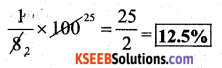b) $$\frac{5}{4}$$
Solution: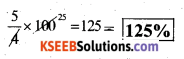c) $$\frac{3}{40}$$
Solution: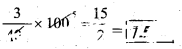d) $$\frac{2}{7}$$
Solution: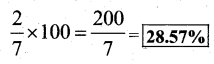Question 2.
Convert the given decimal fractions to per cents.
a) 0.65
Solution:b) 2.1
Solution: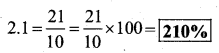c) 0.02
Solution:d) 12.35
Solution:The percent decrease calculator calculates the amount of decrease, in which we would say, “x percent decrease”.

Question 3.
Estimate what part of the figures is coloured and hence find the per cent which is coloured.Solution: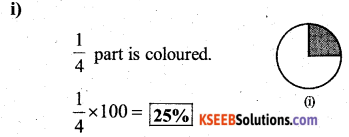∴ In the first figure 25% is coloured.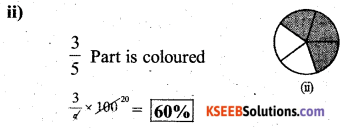∴ in the second figur e 50% Is coloured,.∴ In the third figure 37.5 % is coloured.Question 4.
Find :
a) 15% of 250
Solution: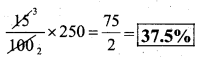b) 1% of 1 hour
Solution: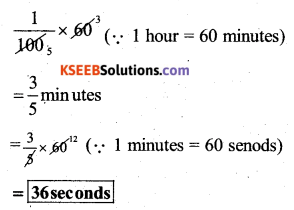c) 20% of ₹ 2500
Solution:d) 75% of ₹ 1 kg
Solution:Question 5.
Find the whole quantity if
a) 5% of it is 600.
Solution:
Let the whole quantity be ‘m’
∴ 5% of m = 600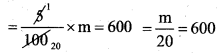∴ The whole quantity is 12,000.

b) 12% of its 1080.
Solution:
Let the whole quantity be ‘m’
∴ 12% of m = Rs. 1080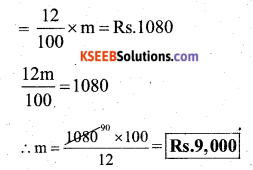∴ The whole amount be Rs. 9.000

c) 40 % of it is 500 km
Solution:
Let the whole quantity be ‘m’
∴ 40% of m = 500 km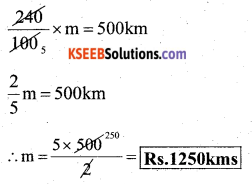∴ The whole amount is 1250 kmsd) 70% of it is 14 minutes
Let the whole quantity be ‘m’∴ The whole amount is 20 minutes

e) 8% of it is 40 litres.
Let the whole quantity be ‘m’∴ The whole amount be 500 litersQuestion 6.
Convert given per cents to decimal fractions and also to fractions in simplest forms:
Solution: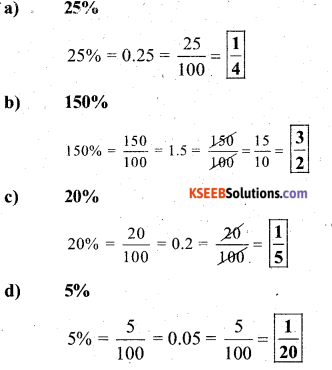Question 7.
In a city, 30% are females, 40% are males and the remaining are children. What percent are children?
Solution:
females = 30%
males = 40%
children = 100 – (30 + 40)
remaining are children = 100 – 70 = 30%
∴ Percentage of children = 30%Percentage difference calculator tool makes the calculation faster, and it displays the difference in the percentage in a fraction of seconds.

Question 8.
Out of 15,000 votes in a constituency, 60% voted. Find the percentage of voters who did not vote. Can you now find how many actually did not vote?
Solution:
Total no. of voters in a constituency } = 15,000
Percentage of voted = 60%
∴ Percentage of voters who did not vote
= 100 – 60 = 40%
Actual number of voters who did not vote} = 40% of 15,0006000 voters who did not vote.

I hope you guys are now clear about how to convert CGPA to percentage in the SPPU mark sheet.

Question 9.
Meeta saves ₹ 400 from her salary. If this is 10% of her salary. What is her salary?
Solution:
Let Meeta’s salary be ‘M’
10% of M is = Rs. 400
∴ M =?∴ Her salary be Rs. 4,000/-Question 10.
A local cricket team played 20 matches in one season. It won 25% of them. How many matches did they win?
Let the total matches won by them be M 25% of 20 = M∴ They win 5 matches.

error: Content is protected !!